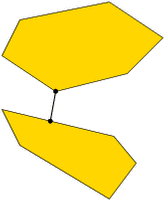CGAL 4.14 - Optimal Distances
User Manual

This chapter describes how to compute the distance between the convex hulls of two given point sets in $$d$$-dimensional Euclidean space (Polytope_distance_d<Traits>). Moreover, it is possible to compute the width of a point set in three dimensions (Width_3<Traits>).The obvious application is collision detection between convex bodies in space. In the spirit of the bounding volume application above, it also makes sense for nonconvex objects: a full intersection test between complicated objects could in a first stage be approximated with the test between the convex hulls of the objects. Only if the hulls intersect, a full intersection test is necessary.

To dampen fears concerning the performance of the distance computation, we want to mention that the convex hulls of the input point sets are not explicitly computed. This avoids a runtime which grows exponentially in $$d$$. In fact, the runtime is almost always linear in the size of the two point sets.